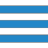﻿ MySQL JOINS Slide Presentation - w3resource# MySQL Joins - slides presentationThis presentation describes MySQL Joins in detail.

## Transcript

What is JOIN in MySQL?

★ A join enable you to retrieve records from two (or more) logically related tables in a single result set.
★ JOIN clauses are used to return the rows of two or more queries using two or more tables that shares a meaningful relationship based on a common set of values.
★ These values are usually the same of column name and datatype.
★ These columns, or possibly a single column from each table, are called the join key or common key.
★ Mostly the join key is the primary key of one table and a foreign key in another table.
★ The join can be performed as long as the data in the columns are matching.

Types of JOIN

★ INNER JOIN
★ LEFT JOIN
★ RIGHT JOIN
★ STRAIGHT_JOIN or STRAIGHT JOIN
★ CROSS JOIN
★ NATURAL JOIN

MySQL INNER JOIN

★ The INNER JOIN is such a JOIN in which all rows can be selected from both participating tables as long as there is a match between the columns.
★ INNER JOIN combines the tables.
★ An INNER JOIN allows rows from either table to appear in the result if and only if both tables meet the conditions specified in the ON clause.

Example : MySQL INNER JOIN

SELECT * FROM table_A
INNER JOIN table_B
ON table_A.A=table_B.A;

MySQL LEFT JOIN

★ The LEFT JOIN fetches all records from the table on the left side of the join statement.
★ If a record from the left table has no matching record in the table on the right side of the join, then the corresponding column from the right table returns a NULL value.

Example : MySQL LEFT JOIN

SELECT * FROM table_A
LEFT JOIN table_B
ON table_A.A=table_B.A;

MySQL RIGHT JOIN

★ The RIGHT JOIN fetched all records from the table on the right side of the join statement, even if the table on the left has no matching record.
★ The columns for unmatched rows from the left table return NULL values.

Example : MySQL RIGHT JOIN

SELECT * FROM table_A
RIGHT JOIN table_B
ON table_A.A=table_B.A;

MySQL STRAIGHT JOIN

★ A STRAIGHT_JOIN scans and combines matching rows ( if specified any condition) which are stored in associated tables otherwise it behaves like an INNER JOIN or JOIN of without any condition.

Example : MySQL STRAIGHT JOIN

SELECT * FROM table_A
STRAIGHT JOIN table_B;

MySQL CROSS JOIN

★ A CROSS JOIN is the complete cross product of two tables.
★ For each record in the first table, all the records in the second table are joined.
★ Creating a potentially huge result set.
★ This command has the same effect as leaving off the join condition.
★ This result set is also known as a Cartesian product.

Example : MySQL CROSS JOIN

SELECT * FROM table_A
CROSS JOIN table_B;

MySQL NATURAL JOIN

★ A NATURAL JOIN is such a join that performs the same task as an INNER or LEFT JOIN, in which the ON or USING clause refers to all columns that the tables to be joined have in common.

Example : MySQL NATURAL JOIN

SELECT * FROM table_A
NATURAL JOIN table_B;

﻿